# 桶排序思想与高频面试题---相邻两数最大插值（maxGap）

2019/05/13 21:30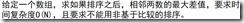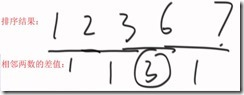N个数准备N+1个桶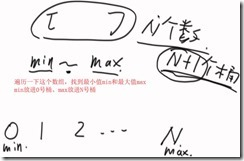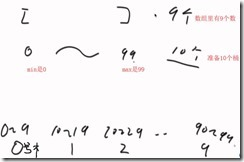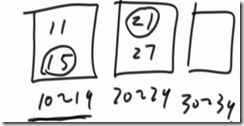15的相邻值，可能来自于11（同一个桶），可能来自于21（相邻桶）

N个数，准备N+1个桶，min放进第0个桶，max放进第N个桶，中间必有一个空桶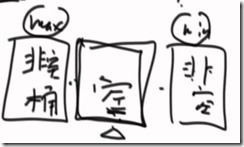（1） 有数字进入某个桶，桶的boolean类型的变量就要从false变为true

（2） 如果是第一次进入桶，那min = max = 这个数

（3） 如果某个数字再次进入了某个桶，就更新这个桶中的min 和max

（4） 然后从1号桶开始（因为0号桶一定非空），如果是空的，跳到下一个桶，如果是非空的，找前一个非空桶。对于每一个非空桶，都找它前一个非空桶的max和当前这个桶的min

（5） min–max记录最大差值

（6） 要求的最大差值一定在其中是最大的，用一个全局变量就可以找到它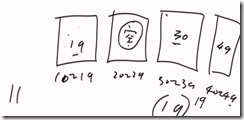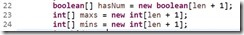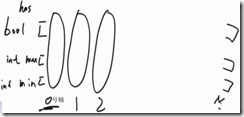import java.util.Arrays;

public class MaxGap {

public static void main(String[] args) {
int[] arr ={0,2,15,23,21,67,88,4,99};//答案44
//        int[] arr ={1,2,3,6,7};//答案3
System.out.println(maxGap(arr));

}

public static int maxGap(int[] arr){
if (arr.length<2 || arr == null) {
return 0;
}
//1.遍历找到数组中的最小值min最大值max
int min = arr;
int max = arr;
for (int i = 1; i < arr.length; i++) {
if (arr[i]<min) {
min = arr[i];
}
if (arr[i]>max) {
max = arr[i];
}
}
System.out.println("min = "+min+" max = "+max);
//2.如果数组中是一串相同的数字，差值为0
if (max == min) {
return 0;
}
//3.构造3个桶
int len = arr.length;
boolean[] hasNum = new boolean[len+1];//数组中的默认值是false
int[] mins = new int[len+1];
int[] maxs = new int[len+1];
//4.重新遍历这个数组，找到每个桶中的max、min
for (int i = 0; i < arr.length; i++) {
//看数组中的每一个数字属于几号桶
int bucketNumber = bucket(arr[i], max, min, len);
System.out.println("第"+i+"个数字在第"+bucketNumber+"号桶");
//桶中无数据
if (!hasNum[bucketNumber]) {
mins[bucketNumber] = arr[i];
maxs[bucketNumber] = arr[i];
hasNum[bucketNumber] = true;
}else {//桶中有数据
mins[bucketNumber] = Math.min(arr[i], mins[bucketNumber]);
maxs[bucketNumber] = Math.max(arr[i], maxs[bucketNumber]);
}

}
System.out.println("每个桶中的最小值mins"+Arrays.toString(mins));
System.out.println("每个桶中的最大值maxs"+Arrays.toString(maxs));
System.out.println(Arrays.toString(hasNum));

//找当前桶的min和前一个非空桶的max
int maxGap = 0;
int lastMax = maxs;//前一个非空桶的max
for(int i=1; i<hasNum.length; i++){
if (hasNum[i]) {
maxGap = Math.max((mins[i] - lastMax), maxGap);
System.out.println("第"+i+"次最大值为 "+ maxGap);
//                if (lastMax - mins[i] > maxGap) {
//                    maxGap = lastMax - mins[i];
//                }
lastMax = maxs[i];
}
}
return maxGap;
}
/**
* 判断一个数字属于几号桶
* @param num  被判断的数字
* @param max    数组中的最大值
* @param min   数组中的最小值
* @param n     数组中的数据量N
*/
public static int bucket(int num, int max, int min,int n){
return (int)(num-min)*(n+1)/(max-min+1);
}

}

0
0 收藏

### 作者的其它热门文章0 评论
0 收藏
0